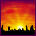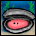# K60 Clock Setup

cancel
Showing results for
Did you mean:
SOLVED

## K60 Clock Setup

5,186 ViewsContributor III

Hi everybody,

I need some help to speed up my core. Before I start to explain my problem - just two things to know:

- I use Keil ARM with RL-RTX for my board, so there is no Processor Expert and MQX available

- I also use the CMSIS header file

okay, according to CMSIS, i have the C-file "system_MK60N512MD100.c" in which is the function SystemInit()

There are also 3 predefinded configurations to setup the clock.

here the code:

`#define CLOCK_SETUP     3/* Predefined clock setups   0 ... Multipurpose Clock Generator (MCG) in FLL Engaged Internal (FEI) mode         Core clock/Bus clock derived from an internal clock source 32.768kHz         Core clock = 47.97MHz, BusClock = 47.97MHz   1 ... Multipurpose Clock Generator (MCG) in PLL Engaged External (PEE} mode         Clock derived from and external crystal 8MHz         Core clock = 48MHz, BusClock = 48MHz   2 ... Multipurpose Clock Generator (MCG) in Bypassed Low Power External (BLPE) mode         Core clock/Bus clock derived directly from external crystal with no multiplication         Core clock = 8MHz, BusClock = 8MHz*/`

`/*----------------------------------------------------------------------------  Define clock source values *----------------------------------------------------------------------------*/#if (CLOCK_SETUP == 0)  #define CPU_XTAL_CLK_HZ           4000000u /* the external crystal or oscillator clock frequency in Hz */  #define CPU_XTAL32k_CLK_HZ      32768u /* the external 32k crystal or oscillator clock frequency in Hz */  #define CPU_INT_SLOW_CLK_HZ   32768u   /* the slow internal oscillator clock frequency in Hz  */  #define CPU_INT_FAST_CLK_HZ     4000000u /* the fast internal oscillator clock frequency in Hz  */  #define DEFAULT_SYSTEM_CLOCK  47972352u /* Default System clock value */#elif (CLOCK_SETUP == 1)  #define CPU_XTAL_CLK_HZ          8000000u /*  the external crystal or oscillator clock frequency in Hz */  #define CPU_XTAL32k_CLK_HZ     32768u   /* the external 32k crystal or oscillator clock frequency in Hz */  #define CPU_INT_SLOW_CLK_HZ  32768u   /* the slow internal oscillator clock frequency in Hz  */  #define CPU_INT_FAST_CLK_HZ    4000000u /* the fast internal oscillator clock frequency in Hz  */  #define DEFAULT_SYSTEM_CLOCK 48000000u /* Default System clock value */ #elif (CLOCK_SETUP == 2)  #define CPU_XTAL_CLK_HZ           8000000u /* the external crystal or oscillator clock frequency in Hz */  #define CPU_XTAL32k_CLK_HZ     32768u   /* the external 32k crystal or oscillator clock frequency in Hz */  #define CPU_INT_SLOW_CLK_HZ   32768u   /* Value  the slow internal oscillator clock frequency in Hz  */  #define CPU_INT_FAST_CLK_HZ     4000000u /* Value of the fast internal oscillator clock frequency in Hz  */  #define DEFAULT_SYSTEM_CLOCK  80000000u /* Default System clock value */#endif /* (CLOCK_SETUP == 2) */`

this is in the function SystemInit()

```  /* System clock initialization */#if (CLOCK_SETUP == 0)  /* Switch to FEI Mode */  /* MCG->C1: CLKS=0,FRDIV=0,IREFS=1,IRCLKEN=1,IREFSTEN=0 */  MCG->C1 = (uint8_t)0x06u;  /* MCG->C2: ??=0,??=0,RANGE=0,HGO=0,EREFS=0,LP=0,IRCS=0 */  MCG->C2 = (uint8_t)0x00u;  /* MCG_C4: DMX32=1,DRST_DRS=1 */  MCG->C4 = (uint8_t)((MCG->C4 & (uint8_t)~(uint8_t)0x40u) | (uint8_t)0xA0u);  /* MCG->C5: ??=0,PLLCLKEN=0,PLLSTEN=0,PRDIV=0 */  MCG->C5 = (uint8_t)0x00u;  /* MCG->C6: LOLIE=0,PLLS=0,CME=0,VDIV=0 */  MCG->C6 = (uint8_t)0x00u;  while((MCG->S & MCG_S_IREFST_MASK) == 0u) { /* Check that the source of the FLL reference clock is the internal reference clock. */  }  while((MCG->S & 0x0Cu) != 0x00u) {    /* Wait until output of the FLL is selected */  }  /* SIM->CLKDIV1: OUTDIV1=0,OUTDIV2=0,OUTDIV3=1,OUTDIV4=1,??=0,??=0,??=0,??=0,??=0,??=0,??=0,??=0,??=0,??=0,??=0,??=0,??=0,??=0,??=0,??=0 */  SIM->CLKDIV1 = (uint32_t)0x00110000u; /* Update system prescalers */#elif (CLOCK_SETUP == 1)/* Switch to FBE Mode */
/* OSC->CR: ERCLKEN=0,??=0,EREFSTEN=0,??=0,SC2P=0,SC4P=0,SC8P=0,SC16P=0 */
OSC->CR = (uint8_t)0x00u;
/* SIM->SOPT2: MCGCLKSEL=0 */
SIM->SOPT2 &= (uint8_t)~(uint8_t)0x01u;
/* MCG->C2: ??=0,??=0,RANGE=2,HGO=0,EREFS=1,LP=0,IRCS=0 */
MCG->C2 = (uint8_t)0x24u;
/* MCG->C1: CLKS=2,FRDIV=3,IREFS=0,IRCLKEN=1,IREFSTEN=0 */
MCG->C1 = (uint8_t)0x9Au;
/* MCG->C4: DMX32=0,DRST_DRS=0 */
MCG->C4 &= (uint8_t)~(uint8_t)0xE0u;
/* MCG->C5: ??=0,PLLCLKEN=0,PLLSTEN=0,PRDIV=3 */
MCG->C5 = (uint8_t)0x03u;
/* MCG->C5: PLLCLKEN=1 */
MCG->C5 |= (uint8_t)0x40u;            /* Enable the PLL */
/* MCG->C6: LOLIE=0,PLLS=0,CME=0,VDIV=0 */
MCG->C6 = (uint8_t)0x00u;
while((MCG->S & MCG_S_OSCINIT_MASK) == 0u) { /* Check that the oscillator is running */
}
while((MCG->S & MCG_S_IREFST_MASK) != 0u) { /* Check that the source of the FLL reference clock is the external reference clock. */
}
while((MCG->S & 0x0Cu) != 0x08u) {    /* Wait until external reference clock is selected as MCG output */
}
/* Switch to PBE Mode */
/* MCG->C1: CLKS=2,FRDIV=0,IREFS=0,IRCLKEN=1,IREFSTEN=0 */
MCG->C1 = (uint8_t)0x82u;
/* MCG->C6: LOLIE=0,PLLS=1,CME=0,VDIV=0 */
MCG->C6 = (uint8_t)0x40u;
/* Switch to PEE Mode */
/* MCG->C1: CLKS=0,FRDIV=0,IREFS=0,IRCLKEN=1,IREFSTEN=0 */
MCG->C1 = (uint8_t)0x02u;
/* MCG->C5: ??=0,PLLCLKEN=0,PLLSTEN=0,PRDIV=3 */
MCG->C5 = (uint8_t)0x03u;
/* MCG->C6: LOLIE=0,PLLS=1,CME=0,VDIV=0 */
MCG->C6 = (uint8_t)0x40u;
while((MCG->S & 0x0Cu) != 0x0Cu) {    /* Wait until output of the PLL is selected */
}
while((MCG->S & MCG_S_LOCK_MASK) == 0u) { /* Wait until locked */
}
/* SIM->CLKDIV1: OUTDIV1=0,OUTDIV2=0,OUTDIV3=1,OUTDIV4=1,??=0,??=0,??=0,??=0,??=0,??=0,??=0,??=0,??=0,??=0,??=0,??=0,??=0,??=0,??=0,??=0 */
SIM->CLKDIV1 = (uint32_t)0x00110000u; /* Update system prescalers */
#elif (CLOCK_SETUP == 2)
...
...```

changing some defines in the second part have no effect...these values have no reference to the other code or files..I just postet for the sake of completeness...

To change clock, I only have to change CLOCK_SETUP from 0 to 1 or 2

0 and 2 are working, 1 unfortunatly not.

I found out, that I have to change the modes from FEI to FBE to PBE  to PEE to get the maximal core-frequenzy (96 MHz)...am I right ?

In uVision, I got the opportunity to manually change the registers -> but I always stuck by swtiching into PEE mode.The debug mode is exiting without an error.

Does anybody have some experience about this all? Or can anybody say why CLOCK_SETUP 1 is not working ?

Regards, Philip

1 Solution
997 ViewsContributor III

A service request gave me a answer:

it is possible to messure the frequency this way, but you have to divide it by 2.

"TRACE_CLKOUT is half of CPU clock"

so its everything correct. Thanks Brun.

7 Replies
997 ViewsContributor I

I am running a bareboard setup and can not get my clocks and PIT to work correctly.

First is there code that sets up the K60 Tower with its 50Mhz OSC?

I need 96Mhz core, 48Mhz bus 24 Mhz flash and 48Mhz USB ciocks.

Could someone also step me through PIT setup..... I set it up now and it runs very slow :smileyhappy:

It seems that my system is running at 96Mhz from a LED toggle program I wrote and put it on scope, it looked like approx 20ns...... but PIT runs from bus clock right?

THanks!

997 ViewsContributor III

Is the PEE mode working on your controller?

I copied the configuration from CMSIS startup - so normaly, it should work :-/

The programm starts directly in debug mode...in all other CLOCL_SETUP modes, I have to start it manually...

I also cannot stop or reset the controller per software.

"Could not stop Cortex-M device! Please check the JTAG cable."

What does this mean?

997 ViewsContributor III

does nobody have any experience? Are you all running your K60 in lowspeed?

Here again some code...

FEI -> FBE

`  /* Switch to FBE Mode */  /* OSC->CR: ERCLKEN=0,??=0,EREFSTEN=0, */  OSC->CR = (uint8_t)0x00u;  /* SIM->SOPT2: MCGCLKSEL=0 TRACECLKSEL=1 */  SIM->SOPT2 = SIM_SOPT2_TRACECLKSEL_MASK;  /* MCG->C2: ??=0,??=0,RANGE=2,HGO=0,EREFS=1,LP=0,IRCS=0 */  MCG->C2 = (uint8_t)0x24u;  /* MCG->C1: CLKS=2,FRDIV=3,IREFS=0,IRCLKEN=1,IREFSTEN=0 */  MCG->C1 = (uint8_t)0x9Au;  /* MCG->C4: DMX32=0,DRST_DRS=0 */  MCG->C4 &= (uint8_t)~(uint8_t)0xE0u;  /* MCG->C5: PLLCLKEN=0,PLLSTEN=0,PRDIV=25(50MHz / 25 = 2MHz)*/  MCG->C5 = (uint8_t)0x18u;  /* MCG->C5: PLLCLKEN=1 */  MCG->C5 |= (uint8_t)0x40u;            /* Enable the PLL */  /* MCG->C6: LOLIE=0,PLLS=0,CME=0,VDIV=0 */  MCG->C6 = (uint8_t)0x00u;  while((MCG->S & MCG_S_OSCINIT_MASK) == 0u) { /* Check that the oscillator is running */  }  while((MCG->S & MCG_S_IREFST_MASK) != 0u) { /* Check that the source of the FLL reference clock is the external reference clock. */  }  while((MCG->S & 0x0Cu) != 0x08u) {    /* Wait until external reference clock is selected as MCG output */  }`

FBE->PBE

`   /* Switch to PBE Mode */  /* MCG->C1: CLKS=2,FRDIV=0,IREFS=0,IRCLKEN=1,IREFSTEN=0 */  MCG->C1 = (uint8_t)0x82u;  /* MCG->C6: LOLIE=0,PLLS=1,CME=0,VDIV=0 */  MCG->C6 = (uint8_t)0x40u;`

PBE->FEI

`  /* Switch to PEE Mode */  /* MCG->C1: CLKS=0,FRDIV=0,IREFS=0,IRCLKEN=1,IREFSTEN=0 */   MCG->C1 = (uint8_t)0x02u;  /* MCG->C5: ??=0,PLLCLKEN=0,PLLSTEN=0,PRDIV=25 */  MCG->C5 = (uint8_t)0x18u;    /* MCG->C6: LOLIE=0,PLLS=1,CME=0,VDIV=50 (2MHz * 48 = 96MHz) */  MCG->C6 = (uint8_t)0x58u;    while((MCG->S & 0x0Cu) != 0x0Cu) {    /* Wait until output of the PLL is selected */  }  while((MCG->S & MCG_S_LOCK_MASK) == 0u) { /* Wait until locked */  }  /* SIM->CLKDIV1: OUTDIV1=0,OUTDIV2=1,OUTDIV3=3,OUTDIV4=1 */  SIM->CLKDIV1 = (SIM_CLKDIV1_OUTDIV1(0) | SIM_CLKDIV1_OUTDIV2(1) | SIM_CLKDIV1_OUTDIV3(3) | SIM_CLKDIV1_OUTDIV4(1));`

Calculation:

50 MHz external reference clock deviced by PRDIV=25  --> 2 MHz input to PLL

2 MHz multiply VDIV=48 -->96 MHz to MCGOUTCLK

Core / System Clock = 96 MHz

Bus Clock = 48 MHz

after this, a simple LED programm should run...but it exit before

SIM->SCGC5    |= (1UL <<  9);        /* Enable Clock to Port A */

PORTA->PCR = (1UL <<  8);        /* Pin is GPIO */
PTA->PDOR = (1 << 28);
PTA->PDDR |= (1 << 28);

...

...

997 ViewsContributor I

That works fine with the K60 TWR:

`/* MCG->C2: ??=0,??=0,RANGE=1,HGO=1,EREFS=1,LP=0,IRCS=0 */  MCG->C2 = MCG_C2_RANGE(1)|MCG_C2_HGO_MASK| MCG_C2_EREFS_MASK;  /* MCG->C1: CLKS=2,FRDIV=2,IREFS=0,IRCLKEN=0,IREFSTEN=0 */  MCG->C1 = MCG_C1_CLKS(2)|MCG_C1_FRDIV(3);      while((MCG->S & MCG_S_OSCINIT_MASK) == 0u) { /* Check that the oscillator is running */  }  while((MCG->S & MCG_S_IREFST_MASK) != 0u) { /* Check that the source of the FLL reference clock is the external reference clock. */  }  while((MCG->S & MCG_S_CLKST_MASK) != 0x08u) {    /* Wait until external reference clock is selected as MCG output */  }    /* MCG->C5: ??=0,PLLCLKEN=0,PLLSTEN=0,PRDIV=1 */  MCG->C5 = MCG_C5_PRDIV(0x17u);   /* MCG->C6: LOLIE=0,PLLS=1,CME=1,VDIV=0 */  MCG->C6 = MCG_C6_CME_MASK|MCG_C6_PLLS_MASK;    while(!(MCG->S & MCG_S_PLLST_MASK)) { /* Check that the oscillator is running */  }  while(!(MCG->S & MCG_S_LOCK_MASK)) { /* Check that the source of the PLL reference clock is the external reference clock. */  }    SIM->CLKDIV1 =  SIM_CLKDIV1_OUTDIV1(0)| SIM_CLKDIV1_OUTDIV2(0)| SIM_CLKDIV1_OUTDIV3(1)| SIM_CLKDIV1_OUTDIV4(3); /* Update system prescalers */  /* MCG->C1: CLKS=2,FRDIV=0,IREFS=0,IRCLKEN=1,IREFSTEN=0 */  MCG->C1 &= ~MCG_C1_CLKS_MASK;  while((MCG->S & MCG_S_CLKST_MASK) != 0x0Cu) {    /* Wait until external reference clock is selected as MCG output */  } `

K60P144M100SF2RM.pdf @ page586

997 ViewsContributor III

`/* MCG->C5: ??=0,PLLCLKEN=0,PLLSTEN=0,PRDIV=1 */  MCG->C5 = MCG_C5_PRDIV(0x17u);`

you mean PRDIV is 24, am I right?

Calculation:

50 MHz / 24 = 2,083333 MHz                PRDIV=24

2,083333 MHz * 24 = 50 MHz               VDIV = 24

Core/System Clock should be 50MHz

A short messurement with oscilloscope (before, I included this code):

`    /* Set the trace clock to the core clock frequency */ SIM->SOPT2 |= SIM_SOPT2_TRACECLKSEL_MASK; /* Enable the TRACE_CLKOUT pin function on PTA6 (alt7 function) */ PORTA->PCR = ( PORT_PCR_MUX(0x7));`

I got 25 MHz output on traceclk or Tower A36 pin...

Jumper J6 is on 1-2 state, so external reference clock is 50MHz

Regards

997 ViewsContributor I

r u shure u can measure the frequency this way? (I also see only 25MHz)

but if I enter 50MHz for SysTick_Config(), and for Uart_init(), the timer and the baudrate is as expectet.

998 ViewsContributor III

A service request gave me a answer:

it is possible to messure the frequency this way, but you have to divide it by 2.

"TRACE_CLKOUT is half of CPU clock"

so its everything correct. Thanks Brun.